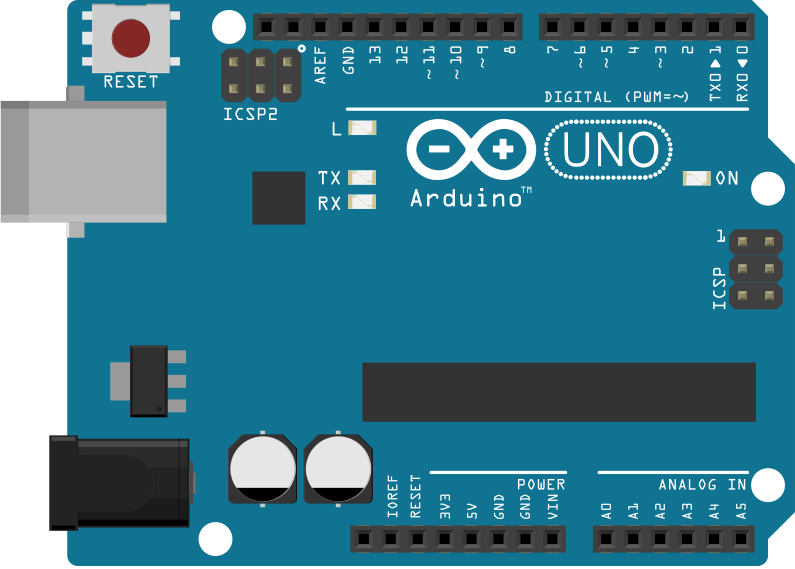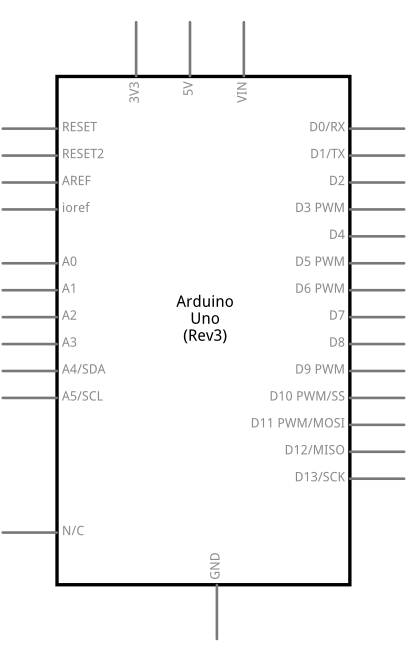EEPROM Iterations

The microcontroller on the Arduino and Genuino boards have 512 bytes of EEPROM: memory whose values are kept when the board is turned off (like a tiny hard drive).

The purpose of this example is to show how to go through the whole EEPROM memory space with different approaches. The code provided doesn’t run on its own but should be used as a surce of code snippets to be used elsewhere.

Hardware Required

• Arduino or Genuino Board

Circuit

There is no circuit for this example.image developed using Fritzing. For more circuit examples, see the Fritzing project page

Schematicsimage developed using Fritzing. For more circuit examples, see the Fritzing project page

Code

/***
eeprom_iteration example.

A set of example snippets highlighting the
simplest methods for traversing the EEPROM.

Running this sketch is not necessary, this is
simply highlighting certain programming methods.

Written by Christopher Andrews 2015
Released under MIT licence.
***/

#include <EEPROM.h>

void setup() {

/***
Iterate the EEPROM using a for loop.
***/

for (int index = 0 ; index < EEPROM.length() ; index++) {

//Add one to each cell in the EEPROM
EEPROM[ index ] += 1;
}

/***
Iterate the EEPROM using a while loop.
***/

int index = 0;

while (index < EEPROM.length()) {

//Add one to each cell in the EEPROM
EEPROM[ index ] += 1;
index++;
}

/***
Iterate the EEPROM using a do-while loop.
***/

int idx = 0;  //Used 'idx' to avoid name conflict with 'index' above.

do {

//Add one to each cell in the EEPROM
EEPROM[ idx ] += 1;
idx++;
} while (idx < EEPROM.length());

} //End of setup function.

void loop() {}